# Orlík hydroelectric plant

The Orlík hydroelectric power plant, built in 1954-1961, consists of four Kaplan turbines. For each of them, the water with a flow rate of Q = 150 m3/s is supplied with a flow rate of h = 70.5 m at full power.
a) What is the total installed power of the power plant at efficiency n = 87%?
b) For the fast charging of electric vehicles overnight, power consumption up to P0 = 11kW is required. How many would electric cars cover power plant power?
c) How many days of full power operation corresponds to the delivered energy E = 398GWh

Correct result:

P =  361.018 MW
n =  32820
d =  45.9

#### Solution:

$h=70.5 \ \text{m} \ \\ Q=150 \ \text{m}^3\text{/s} \ \\ p=87/100=\dfrac{ 87 }{ 100 }=0.87 \ \\ n=4 \ \\ g=9.81 \ \text{m/s}^2 \ \\ w=1000 \ \text{kg/m}^3 \ \\ \ \\ E=m \cdot \ g \cdot \ h \ \\ P_{1}=Q \cdot \ w \cdot \ g \cdot \ h=150 \cdot \ 1000 \cdot \ 9.81 \cdot \ 70.5=103740750 \ \text{W} \ \\ P_{11}=P_{1}/10^6=103740750/10^6 \doteq 103.7408 \ \text{MW} \ \\ P=p \cdot \ n \cdot \ P_{11}=0.87 \cdot \ 4 \cdot \ 103.7408=361.018 \ \text{MW}$
$P_{0}=11/1000=\dfrac{ 11 }{ 1000 }=0.011 \ \text{MW} \ \\ n=P/ P_{0}=361.0178/ 0.011=32820$
$E=398 \ \text{GWh} \ \\ \ \\ P_{2}=P/1000=361.0178/1000 \doteq 0.361 \ \text{GW} \ \\ d=E/(24 \cdot \ P_{2})=398/(24 \cdot \ 0.361)=45.9$We would be very happy if you find an error in the example, spelling mistakes, or inaccuracies, and please send it to us. We thank you!Tips to related online calculators
Do you know the volume and unit volume, and want to convert volume units?
Tip: Our Density units converter will help you with the conversion of density units.
Do you want to convert mass units?
Do you want to convert velocity (speed) units?
Do you want to convert time units like minutes to seconds?

## Next similar math problems:

• A carA car weighing 1.05 tonnes driving at the maximum allowed speed in the village (50 km/h) hit a solid concrete bulkhead. Calculate height it would have to fall on the concrete surface to make the impact intensity the same as in the first case!
• Sea on the Moon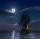Assume that the Moon has sea, the same composition as on the Earth (has same density of salt water). Calculate dive of boat floating in the sea on the Moon, when on Earth has dive 3.6 m. Consider that the Moon has 6.5-times smaller gravitational accelerat
• Chained winch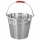When digging a well, a chained winch is used to balance the material. An empty shackle weighs 8 kg, a full 64 kg. What force must the worker exert on the crank handle when pulling the shackle with a uniform movement from a depth of 5 m, if the shaft diame
• Skid friction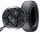Find the smallest coefficient of skid friction between the car tires and the road so that the car can drive at a 200 m radius at 108 km/h and does not skid.
• Angled cyclist turnThe cyclist passes through a curve with a radius of 20 m at 25 km/h. How much angle does it have to bend from the vertical inward to the turn?
• Accelerated motion - mechanicsThe delivery truck with a total weight of 3.6 t accelerates from 76km/h to 130km/h in the 0.286 km long way. How much was the force needed to achieve this acceleration?
• Forces on earth directionsA force of 60 N [North] and 80 N [East] is exerted on an object wigth 10 kg. What is the acceleration of the object?
• Hydrostatic forceWhat hydrostatic force is applied to an area of 30 cm2 in water at a depth of 20 m? (Water density is 1000 kg/m3)
• FlywheelFlywheel turns 450 rev/min (RPM). Determine the magnitude of the normal acceleration of the flywheel point which are at a distance of 10 cm from the rotation axis.
• Shot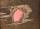Shot with a mass 43 g flying at 256 m/s penetrates into the wood to a depth 25 cm. What is the average force of resistance of wood?
• CarAt what horizontal distance reaches the car weight m = 753 kg speed v = 74 km/h when the car engine develops a tensile force F = 3061 N. (Neglect resistance of the environment.)
• Brick wallWhat is the weight of a solid brick wall that is 30 cm wide, 4 m long and 2 m high? The density of the brick is 1500 kg per cubic meter.
• Brass sphereFind the weight of a brass ball with an outer radius of 12 cm, a wall thickness of 20 mm if the density of the brass is 8.5 g/cm3.
• The water barrel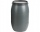The water barrel weighs 122 kg. If we pour 75% of the water out of it, it will weigh 35 kg. What is the weight of the barrel?
• Two teas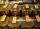A mixture weighing 10 kg was made from two types of tea. The price of tea was 160 CZK/kg and second tea 170 CZK/kg. The price of the mixture is 166 CZK/kg. How many kilograms of each type of tea had to be mixed?
• Copper wireWhat is the mass of 500 m of copper wire with a diameter of 1 mm, if ρ = 8.9 g/cm ^ 3?
• Water reservoir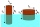What is the weight of a metal reservoir - cylinder with a diameter of 2 m and a length 8 m, if 1 m2 of sheet metal weighs 100 kg?